﻿

# 大数加减乘除，一文彻底搞定

2022-03-21 20:51栏目：互联网
TAG：【CSDN 编者按】最近大数运算频频出现在面试中，在代码编写的过程中也经常涉及比较大的数的运算，当它们超出程序基本类型所能表示的范围时，就会造成溢出，整形一般的加减乘除已经无法实现了，这时候需要自己写一个程序来实现。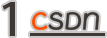## 前言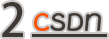## 大数加法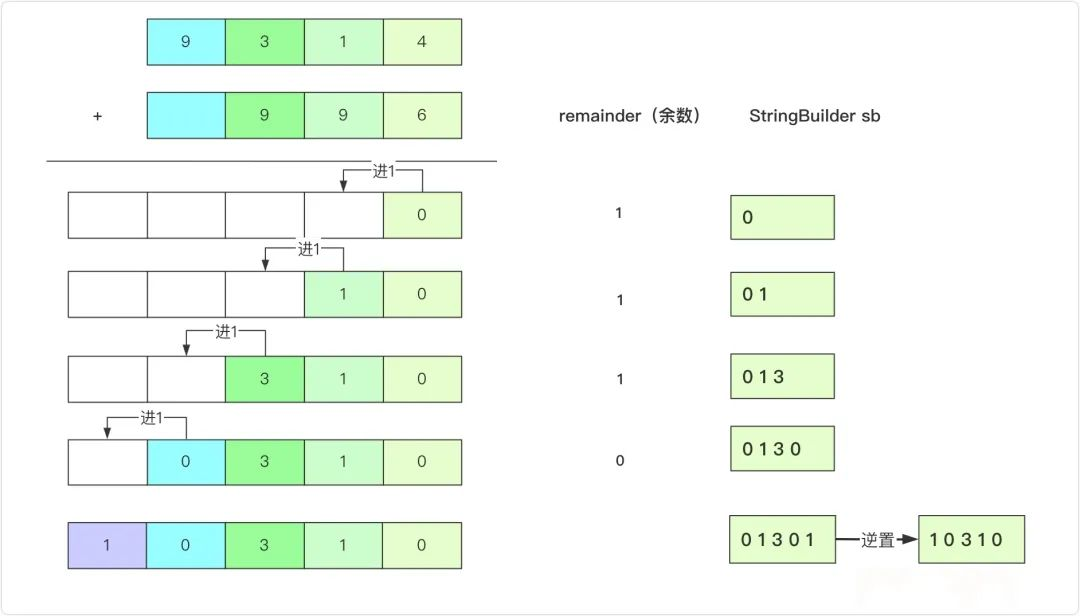public String addStrings(String num1, String num2) {
// ：bigsai 欢迎你的关注
int len1=num1.length-1,len2=num2.length-1;
char ch1=num1.toCharArray;
char ch2=num2.toCharArray;

StringBuilder sb=new StringBuilder;
int remainder =0;//计算余数
while (len1>=0||len2>=0)
{
int n1=len1>=0?(ch1[len1--]-0):0;
int n2=len2>=0?(ch2[len2--]-0):0;
int num=n1+n2+remainder;//求和对应数字
remainder=num/10;//是否进位
sb.append(num%10);// 添加到结果字符串中
}

if(remainder>0)//是否还需要进位
{
sb.append(remainder);
}
//反装即为结果
return sb.reverse.toString;
}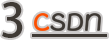## 大数减法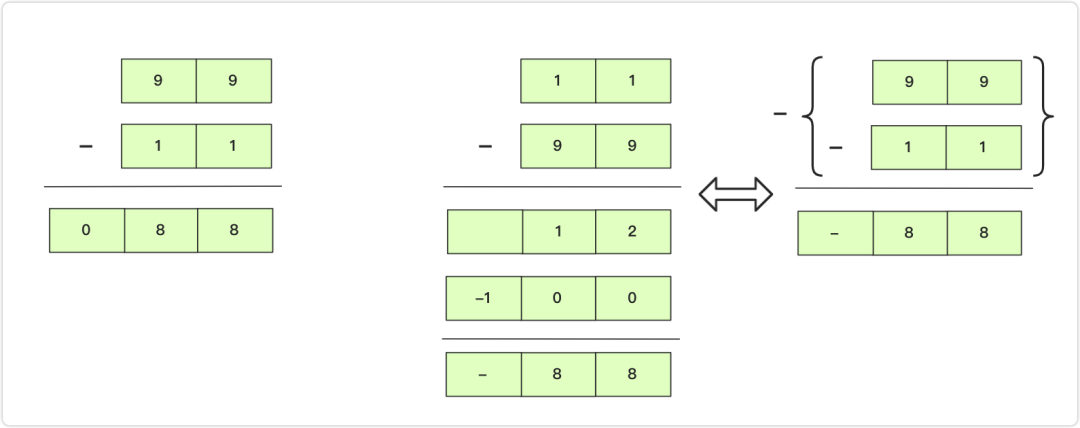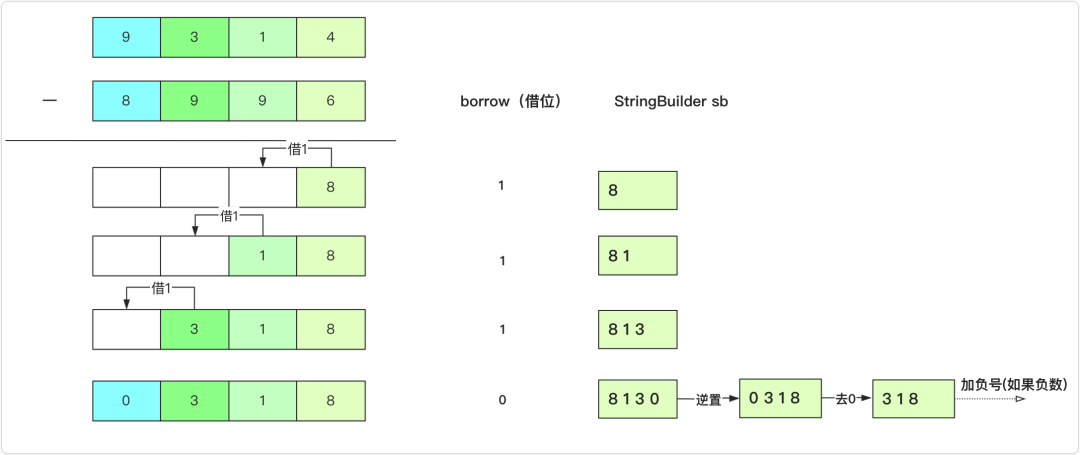public static boolean compare(String num1,String num2)
{
if(num1.length<num2.length)
return false;
else if(num1.length>num2.length)
return true;
else
return num1.compareTo(num2)>0;
}
public static String subtractString(String num1,String num2) {
char sign=+;//正负号
//让num1>num2 如果num1<num2 那么结果就是—(num2-num1)
//可以先将num1和num2交换和前面情况统一
if(!compare(num1,num2))
{
sign=-;
String team = num2;
num2 = num1;
num1 = team;
}
int len1=num1.length-1;
int len2=num2.length-1;

char ch1 = num1.toCharArray;
char ch2 = num2.toCharArray;
StringBuilder sb=new StringBuilder;
int borrow=0;//借位
while (len1>=0||len2>=0)
{
int n1=len1>=0?(ch1[len1--]-0):0;
int n2=len2>=0?(ch2[len2--]-0):0;

int num=n1-n2-borrow;
borrow=0;
if(num<0)//需要向前借位
{
borrow=1;
num+=10;
}
sb.append(num);
}

sb=sb.reverse;//需要先翻转
int index = 0;//去掉前面没用的’0‘
while (index<sb.length&&sb.charAt(index) == 0)
{
index++;
}
//如果两个数相同 直接返回"0"
if(index==sb.length)
return "0";
if(sign==+)//如果正数
return sb.substring(index);
else return sign+sb.substring(index);//负数需要返回
}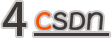## 大数乘法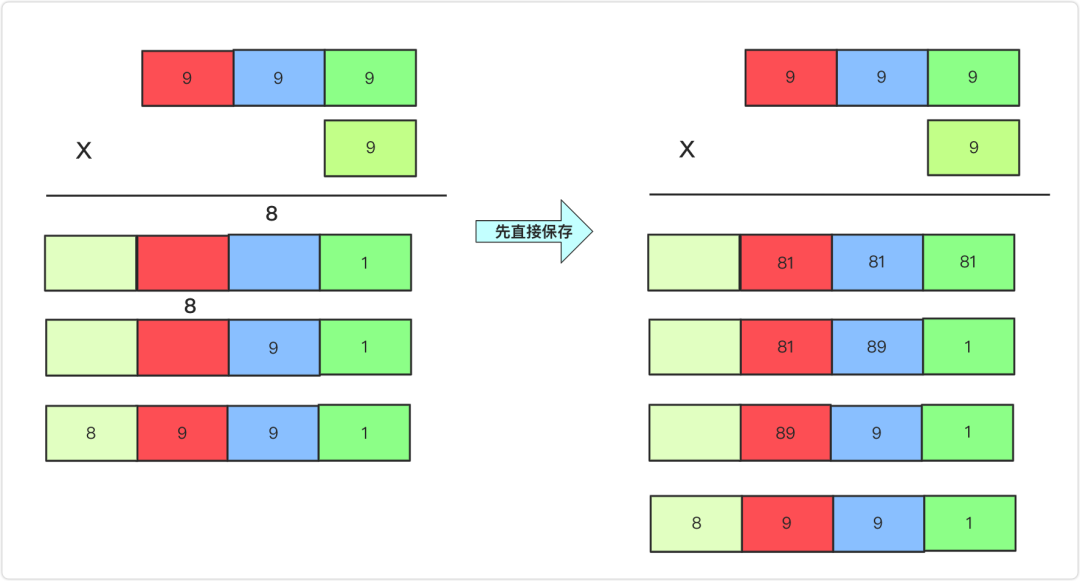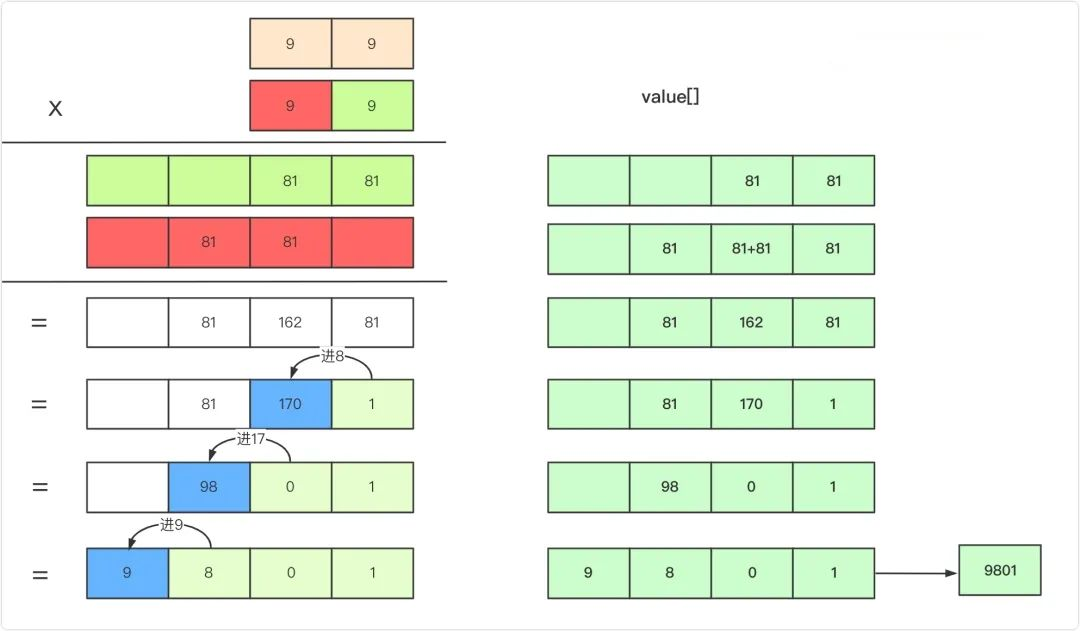a+b大小就够了，怎么分析呢？其中一个a不变。另一个b变成最小b+1数字即十的倍数，那么这样在相乘的时候也不过是a+b长度，所以这里a+b长度就够了。

public String multiply(String num1, String num2) {
if("0".equals(num1)||"0".equals(num2))return "0";
char a=num1.toCharArray;
char b=num2.toCharArray;

int value=new int[a.length+b.length];

for(int i=a.length-1;i>=0;i--)
{
for(int j=b.length-1;j>=0;j--)
{
int index=a.length-1-i+b.length-1-j;
value[index]+=(a[i]-0)*(b[j]-0);
}
}
for(int i=0;i<value.length-1;i++)
{
value[i+1]+=value[i]/10;
value[i]=value[i]%10;
}
int index=value.length-1;
while(value[index]==0)
{index--;}
StringBuilder sBuilder=new StringBuilder;
while (index>=0) {
sBuilder.append(value[index--]);
}
return sBuilder.toString;
}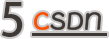## 大数除法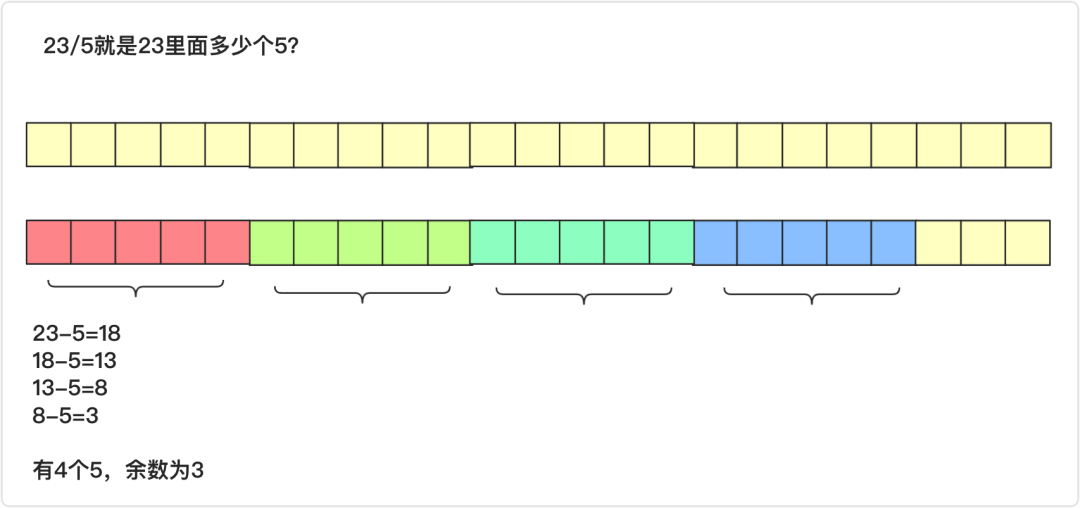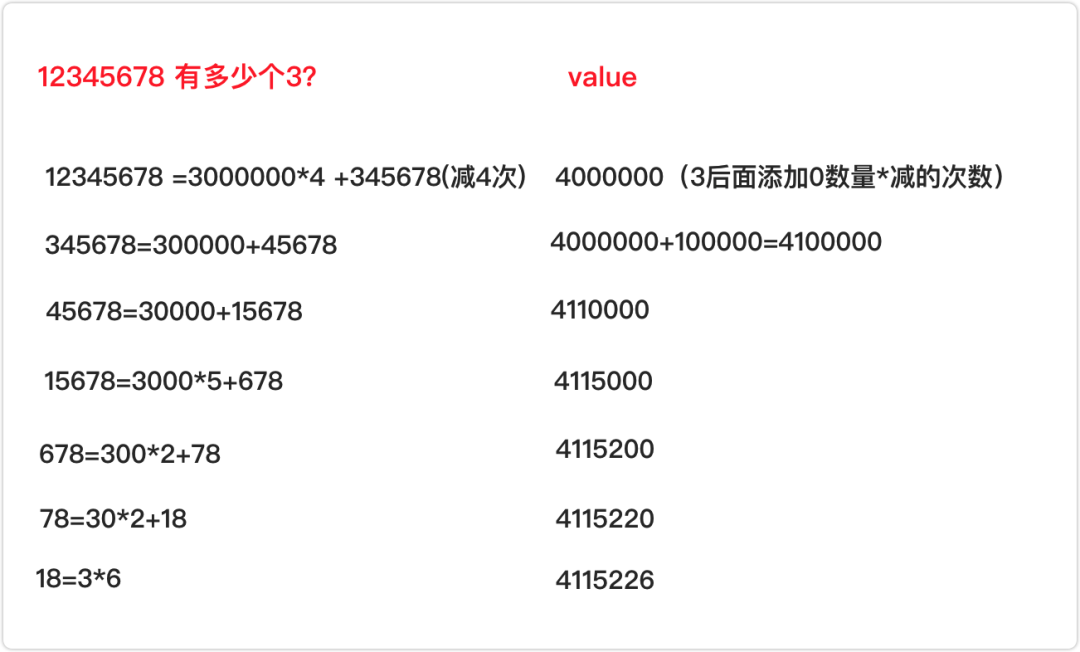//假设 num1>num2
public static String divideString(String num1,String num2)
{
String value="0";//结果
while (compare(num1,num2))
{
StringBuilder sbTeam=new StringBuilder(num2);//用这个往后面不断加0 和num做减法
StringBuilder sbCount=new StringBuilder("1");//次数 可能很大
int subLen=num1.length-num2.length;//统计大概要加几个零
for(int i=0;i<subLen;i++)
{
sbTeam.append(0);
sbCount.append(0);
}
//如果0 加多了 那么要删一个 类似"12300" / "23" "12300"比"23000"小
//所以要 "2300" 对应比"23"扩大 "100"倍数，每减一次"2300" 则结果加"100"
if(!compare(num1,sbTeam.toString))
{
sbTeam=sbTeam.deleteCharAt(sbTeam.length-1);
sbCount=sbCount.deleteCharAt(sbCount.length-1);
}
// 一直能减的时候
while (compare(num1,sbTeam.toString))
{
num1=subtractString(num1,sbTeam.toString);
}
}
return value;
}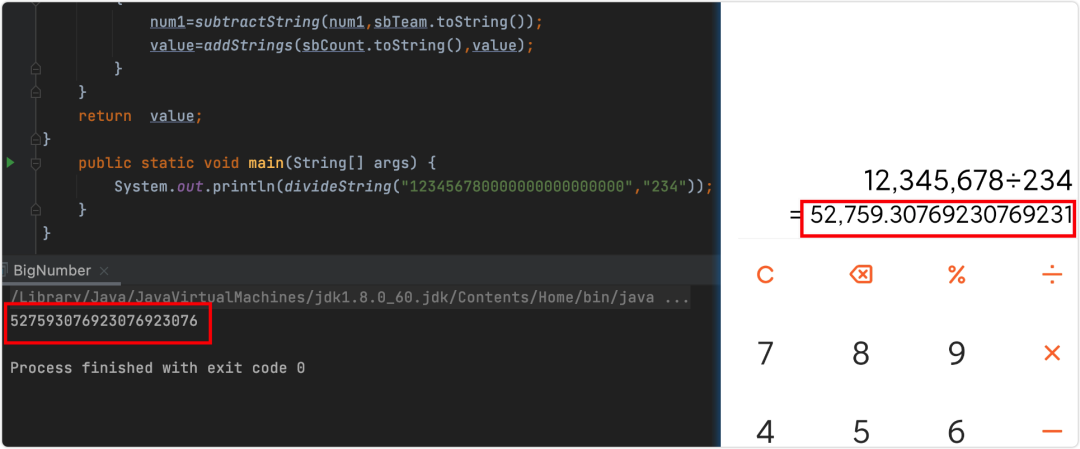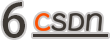## 番外篇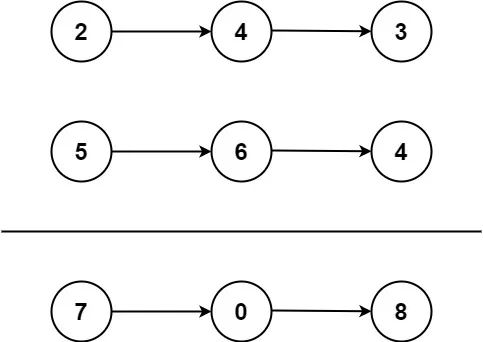img

0 &lt;= Node.val &lt;= 9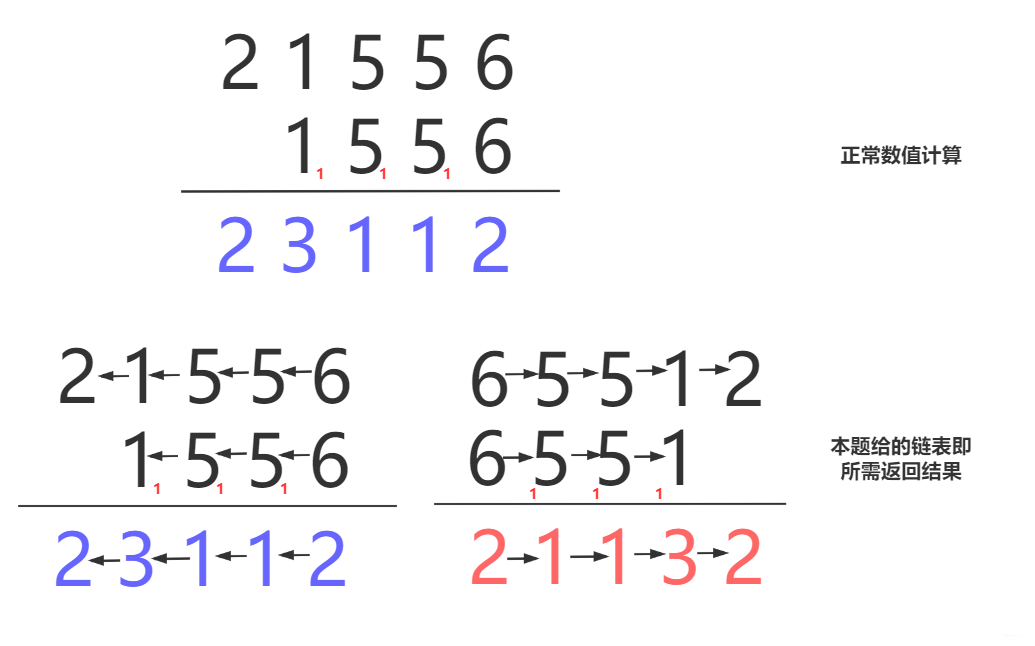//更简洁的写法
public ListNode addTwoNumbers(ListNode l1, ListNode l2) {
ListNode node=new ListNode(0);
ListNode team=node;
int jin=0;//进位
while(l1!=||l2!=)
{
int num=jin;
if(l1!=)
{
num+=l1.val;l1=l1.next;
}
if(l2!=)
{
num+=l2.val;l2=l2.next;
}
jin=num/10;
num%=10;
team.next=new ListNode(num);
team=team.next;

}
if(jin!=0)team.next=new ListNode(jin);
return node.next;
}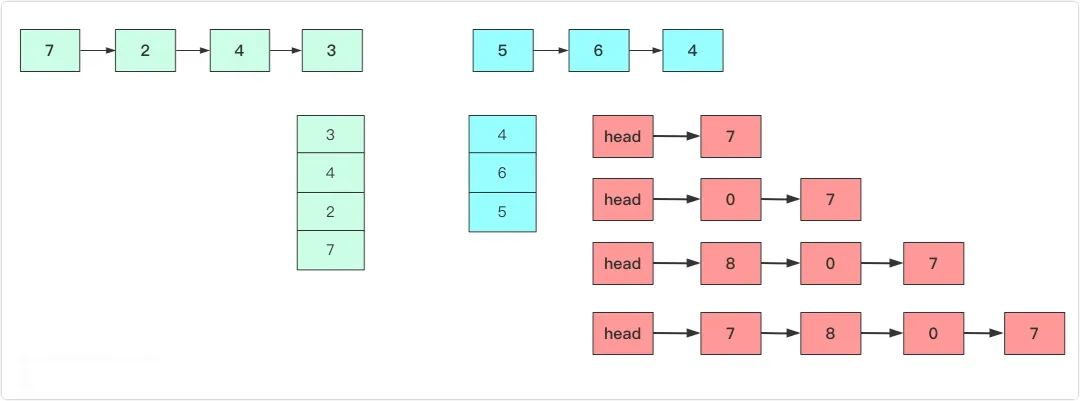/**
* public class ListNode {
* int val;
* ListNode next;
* ListNode(int x) { val = x; }
* }
*/
class Solution {
public ListNode addTwoNumbers(ListNode l1, ListNode l2) {
Stack<Integer>stack1=new Stack<>;
Stack<Integer>stack2=new Stack<>;
while (l1!=)
{
l1=l1.next;
}
while (l2!=)
{
l2=l2.next;
}
ListNode val=new ListNode(0);//带头结点
{
if(!stack1.isEmpty)
num+=stack1.pop;
if(!stack2.isEmpty)
num+=stack2.pop;
num=num%10;
//链表头插
ListNode node=new ListNode(num);
node.next=val.next;
val.next=node;
}
return val.next;
}
}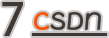## 结语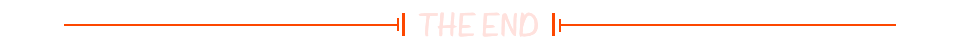4月23日晚8点，欢迎来到CSDN直播间，与资深行业专家雷明老师一起探讨机器学习所必须掌握的数学知识。

﻿